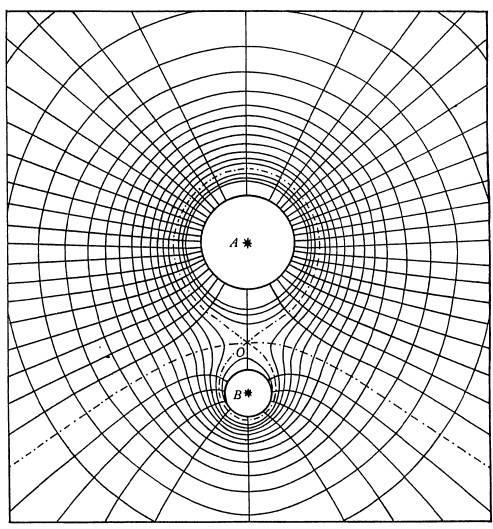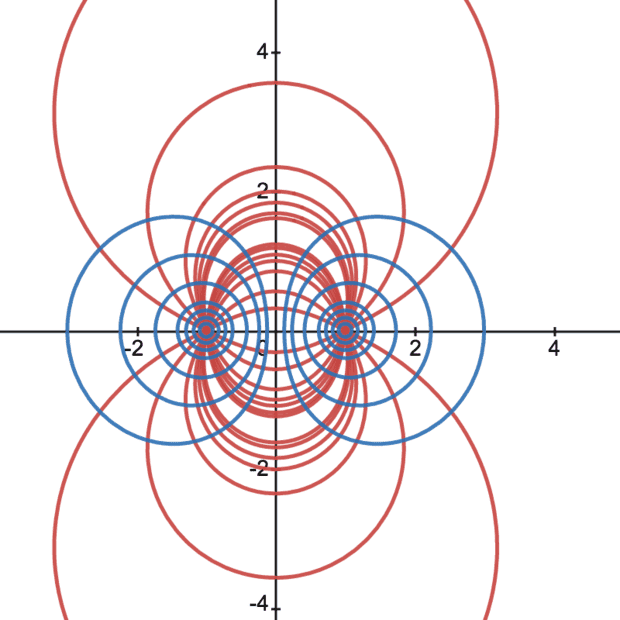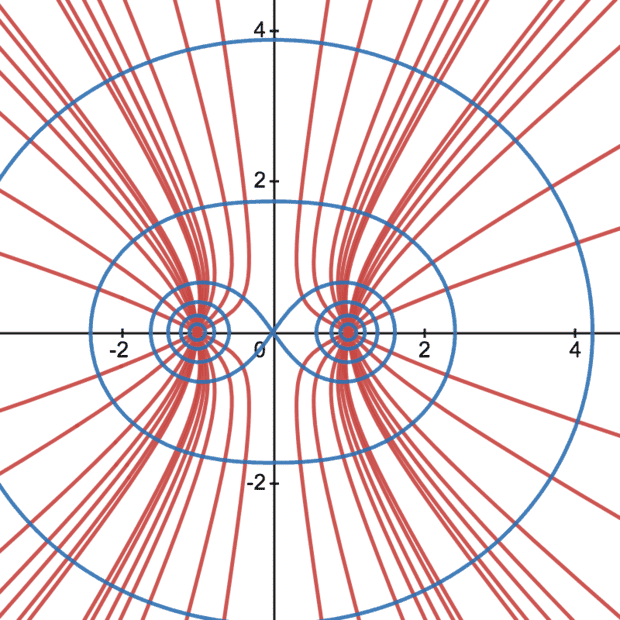# A problem in graphing electric field lines

patric44
Homework Statement:
a problem in Graphing electric field lines
Relevant Equations:
dy/dx = Ey/Ex
hi guys
our instructor asked us to try to graph the projection of the electric field intensity at a certain point p(x,y) , for two charges q+-q located
at (-a,0) , (a,0), Now starting with the equation
$$\frac{dy}{dx} = \frac{E_{y}}{E_{x}}$$
after transforming this equation I got
$$\frac{du}{(1+u^{2})^{3/2}}=\frac{dv}{(1+v^{2})^{3/2}}$$

where $u = (x+a)/y$ , $v = (x-a)/y$

my question is how would I plot the solution of this equation to get the graph of the electric field?

Homework Helper
Gold Member
Do you know how to find orthogonal trajectories? If yes, find the orthogonal trajectories to the equipotential lines of the two charges. If no, look up how to do it on the internet. There are many references. Here is one with examples.

•berkeman and patric44
patric44
so basically i have to solve this equation to get the electric field ?
$$\frac{du}{dv} = \frac{(v^2+1)^{3/2}}{(u^2+1)^{3/2}}$$
another question : how to plot these family of curves say in Matlab

Gold Member
Relevant Equations:: dy/dx = Ey/Ex

after transforming this equation I got
You may integrate the both sides of this equation to get u, v relation. Substitution u, v = tan ##\theta## seems to work.

Gold Member
Homework Statement:: a problem in Graphing electric field lines
Relevant Equations:: dy/dx = Ey/Ex

hi guys
our instructor asked us to try to graph the projection of the electric field intensity at a certain point p(x,y) , for two charges q+-q located
at (-a,0) , (a,0), Now starting with the equation
$$\frac{dy}{dx} = \frac{E_{y}}{E_{x}}$$
after transforming this equation I got
$$\frac{du}{(1+u^{2})^{3/2}}=\frac{dv}{(1+v^{2})^{3/2}}$$

where $u = (x+a)/y$ , $v = (x-a)/y$

my question is how would I plot the solution of this equation to get the graph of the electric field?
Please define what you mean by the projection of the electric field intensity at a certain point p(x,y) because the actual electric field of two point charges is easy to write down. And what does it have to do with ##\frac{dy}{dx} = \frac{E_{y}}{E_{x}}##?

Homework Helper
Gold Member
so basically i have to solve this equation to get the electric field ?
$$\frac{du}{dv} = \frac{(v^2+1)^{3/2}}{(u^2+1)^{3/2}}$$
another question : how to plot these family of curves say in Matlab
How are ##u## and ##v## related to ##x##, ##y## and ##a##?

patric44
You may integrate the both sides of this equation to get u, v relation. Substitution u, v = tan ##\theta## seems to work.
My confusion is how to plot the family of curves after integrating both sides.

patric44
Please define what you mean by the projection of the electric field intensity at a certain point p(x,y) because the actual electric field of two point charges is easy to write down. And what does it have to do with ##\frac{dy}{dx} = \frac{E_{y}}{E_{x}}##?I wish to get something similar to this graph after solving
$$\frac{dy}{dx}=\frac{ \frac{kq\left(x-a\right)}{\left[\left(x-a\right)^2+y^2\right]^{3/2}}\pm \frac{kq\left(x+a\right)}{\left[\left(x+a\right)^2+y^2\right]^{3/2}}}{\frac{kqy}{\left[\left(x-a\right)^2+y^2\right]^{3/2}}\pm\frac{kqy}{\left[\left(x+a\right)^2+y^2\right]^{3/2}}}$$
this is the equation before the transformation
$$u=\frac{x+a}{y}, v=\frac{x-a}{y}.$$

patric44
How are ##u## and ##v## related to ##x##, ##y## and ##a##?
Through the transformation
$$u=\frac{x+a}{y}, v=\frac{x-a}{y}$$

Gold Member
My confusion is how to plot the family of curves after integrating both sides.
Thus got u-v relation is rewritten as x-y relation , I expect.

patric44
Thus got u-v relation is rewritten as x-y relation , I expect.
Yes I tried that, but it doesn't look like the graph shown above! Also, I am trying to figure some way to graph an implicit family of curves using MATLAB or Python.

Gold Member
Could you show us your integral result of u, v functions ?　That seems not so much complicated for writing down.

Last edited:
Gold Member
It seems to me you are not solving for the electric field, you are trying to plot the field lines and lines of equal potential. Is that correct?

Gold Member
View attachment 290951
I wish to get something similar to this graph after solving
$$\frac{dy}{dx}=\frac{ \frac{kq\left(x-a\right)}{\left[\left(x-a\right)^2+y^2\right]^{3/2}}\pm \frac{kq\left(x+a\right)}{\left[\left(x+a\right)^2+y^2\right]^{3/2}}}{\frac{kqy}{\left[\left(x-a\right)^2+y^2\right]^{3/2}}\pm\frac{kqy}{\left[\left(x+a\right)^2+y^2\right]^{3/2}}}$$
this is the equation before the transformation
$$u=\frac{x+a}{y}, v=\frac{x-a}{y}.$$
I still don't see where you got this formula ##\frac{dy}{dx}= \frac{E_y}{E_x}## and what it represents. You already know the electric field everywhere so aren't you merely trying to plot the field knowing the strength at each point? It seems to me there are an infinite number of lines you could plot so how are you going to select which ones?

Last edited:
Gold Member
I still don't see where you got this formula dydx=EyEx and what it represents.
Say line element (dX,dY) is perpendicular to E-field their inner product is zero.
$$E_xdX+E_ydY=0$$
$$dY/dX=-E_x/E_y$$
The lines perpendicular to (dX, dY), so tangent to E everywhere on the line, has tangent of minus reciprocal of the above
$$dy/dx=E_y/E_x$$

•Delta2, PhDeezNutz and bob012345
Gold Member
•BvU
Gold Member
Substitution u, v = tan θ seems to work.
$$\int \frac{du}{(1+u^2)^{3/2}}= \int \frac{\sec^2\theta\ d\theta}{\sec^3\theta}$$

Last edited:
Gold Member
$$\int \frac{du}{(1+u^2)^{3/2}}= \int \frac{\sec^2\theta\ d\theta}{\sec^3\theta}$$
I think one can plot families of curves for like and unlike charges by assuming values for ##\frac{dy}{dx}##.•PhDeezNutz